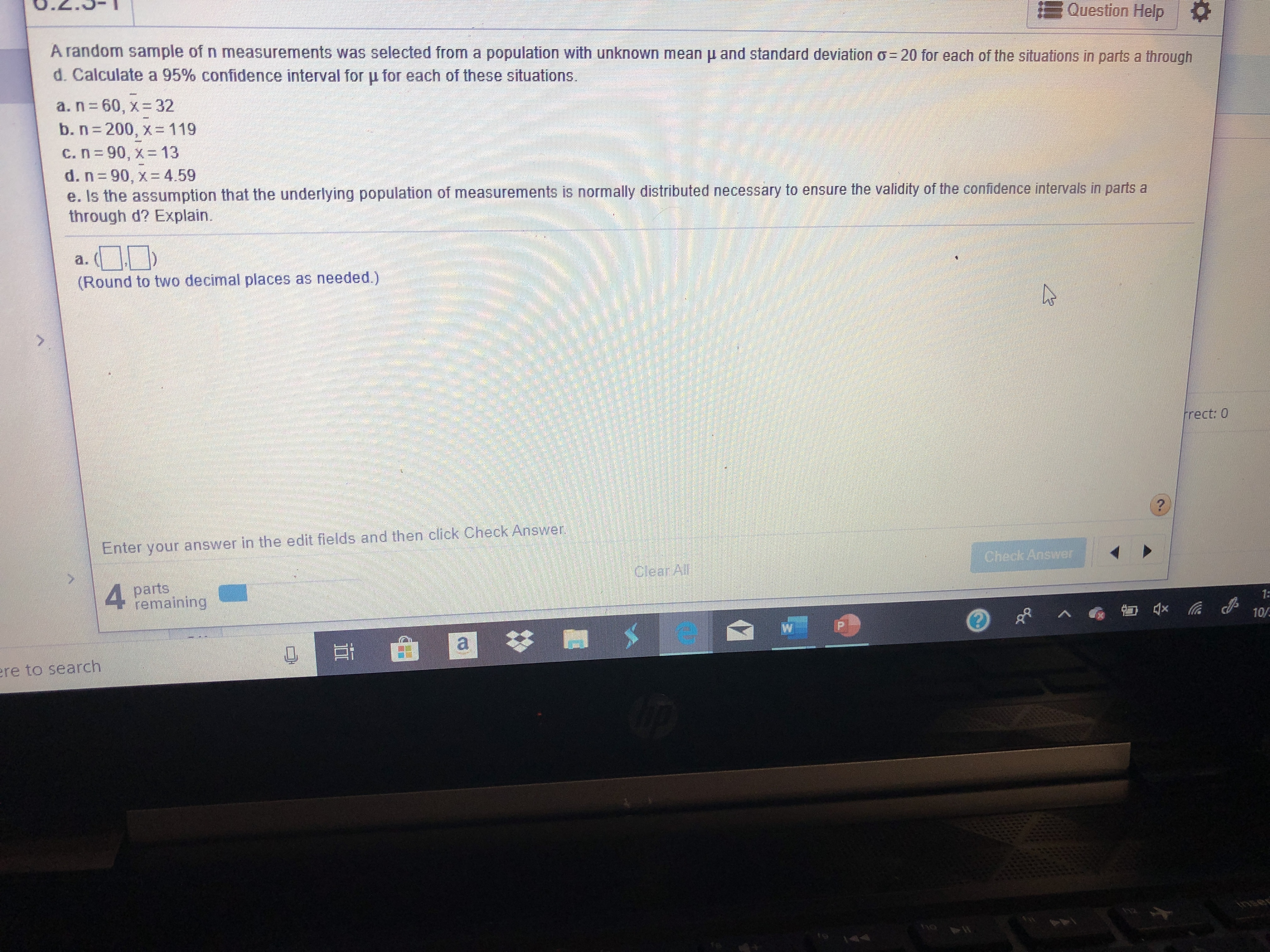# Question Help A random sample of n measurements was selected from a population with unknown mean u and standard deviation o- 20 for each of the situations in parts a through d. Calculate a 95% confidence interval for u for each of these situations. a. n 60, x= 32 b. n 200, x=119 C. n= 90, x=13 d. n 90, x 4.59 e. Is the assumption that the underlying population of measurements is normally distributed necessary to ensure the validity of the confidence intervals in parts a through d? Explain. a. (Round to two decimal places as needed.) frect: 0 ? Enter your answer in the edit fields and then click Check Answer Check Answer Clear Al 1= temaining 10/ W a re to search asui 44

Questionhelp_outlineImage TranscriptioncloseQuestion Help A random sample of n measurements was selected from a population with unknown mean u and standard deviation o- 20 for each of the situations in parts a through d. Calculate a 95% confidence interval for u for each of these situations. a. n 60, x= 32 b. n 200, x=119 C. n= 90, x=13 d. n 90, x 4.59 e. Is the assumption that the underlying population of measurements is normally distributed necessary to ensure the validity of the confidence intervals in parts a through d? Explain. a. (Round to two decimal places as needed.) frect: 0 ? Enter your answer in the edit fields and then click Check Answer Check Answer Clear Al 1= temaining 10/ W a re to search asui 44 fullscreen

### Want to see this answer and more?

Experts are waiting 24/7 to provide step-by-step solutions in as fast as 30 minutes!*